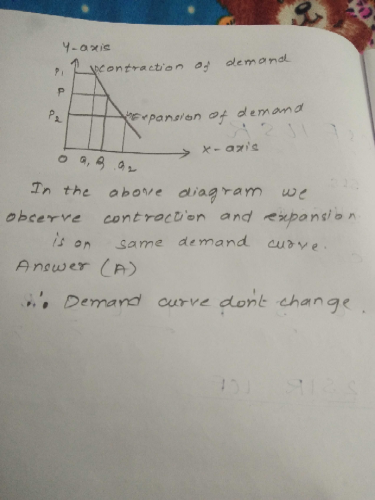Courses

# In expansion and contraction of demand ______a)Demand curve remains unchangedb)Demand curve changesc)Slope of the demand curve changesd)Both  (a) & (b) aboveCorrect answer is option 'B'. Can you explain this answer? Related Test: Test: Theory Of Demand- 2

## CA Foundation QuestionSuraksha Pahwa Jun 15, 2018
When as a result of decrease in price quantity demanded increases, it is expansion of demand and when as a result of increase in price, quantity demanded decreases, it is contraction of demand. it renders the demand curve unchanged but the slope of demand curve changes.Goplar Ajay Dec 17, 2018DAs per law of demand, expansion and contraction occurs along the same demand curve, whereas increase or decrease of demand curve causes the demand curve to change.
SO CORRECT ANSWER IS OPTION AShriyanshu Keshari Aug 13, 2018
Yes when changes take place it goes right side or left sideDashvanth Kumar Dec 12, 2018
Expansion or contraction of demand takes place due to the changes occurred in price only which is generally known as change in quantity demanded in such cases there will be a movement along the same demand curve and the demand curve will not change so option A is the correct answer and not option DChhavi Parakh Aug 01, 2020
As per law of demand if the demand curve satisfies the principle of ceterus paribus(only price changes others things remain constant) then expansion and contraction appear to happen
If the price decreases the quantity demanded increases which lead to expansion in the demand curve and vice versa
If the principle of ceterus paribus does not apply to the demand curve it leads to increase or decrease in demand curve